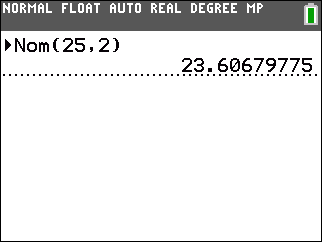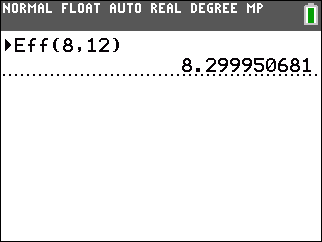# Knowledge Base

## Solution 34792: Computing Nominal and Effective Interest Rates on the TI-83 Plus and TI-84 Plus Family of Graphing Calculators.

### How do I compute the nominal and effective interest rates on the TI-83 Plus and TI-84 Plus family of graphing calculators?

The examples below will demonstrate how to calculate nominal and effective interest rates.

Use the ►Nom() function to compute the nominal interest rate. The syntax for this function is ►Nom(effective rate, compounding periods).

Use the ►Eff() function to compute the effective interest rate. The syntax is ►Eff(nominal rate, compounding periods).

To access these functions, press [APPS] [ENTER] and select [B: ►Nom(] or [C:►Eff(].

Examples:

Find the nominal rate of 25 effective rate with 2 compounding periods:

1) Press [APPS] .
2) Press [ALPHA] [B].
3) Press [(]   [,]  [)].
4) Press [ENTER].

The result is 23.60679775Find the effective rate of 8 with 12 compounding periods:

1) Press [APPS] .
2) Press [ALPHA] [C].
3) Press [(]  [,]   [)].
4) Press [ENTER].

The result is 8.299950681Please see the TI-83 Plus and TI-84 Plus Family guidebooks for additional information.

Last updated: 6/15/2023